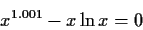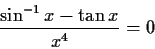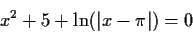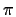WITNESS'' PROBLEMS AND NEW ALGORITHMS

These are problems which are difficult to solve and reveal shortcomings of most of the current methods and systems.

1.For positive x, this equation has two solutions, one small, the other one huge in magnitude:   x= 2.721005...,  0.794138... x103960.

2.This problem and the next were proposed by Kahan. For positive x, this equation has one solution, x=0.999906... This value is too close to 1, the limit where the function can be evaluated without going into the complex plane.

3.For positive x, this equation has two roots very close to, x=3.1415923... and x=3.1415930.... The convergence interval for such roots is extremely narrow, and most methods, even when started close to the roots, will diverge away from them.

4. sin(x) = x/100

This equation has 63 real roots in the interval -96.1<x<96.1. Any good iterator should easily converge to any root given a suitable starting guess.